# Integration by filaments or integration by strate?

Amaelle
Homework Statement:
look at the image
Relevant Equations:
cylindrical coordinates
integration by strates
integration by filaments
Greetings
While solving the following exercice, ( the method used is the integration by filaments and I have no problem doing it this way)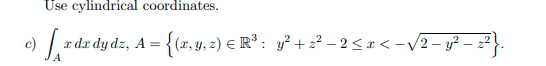here is the solution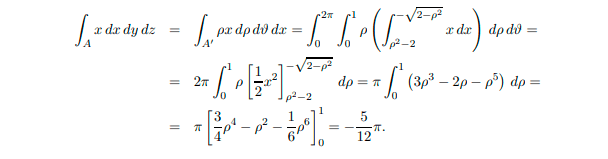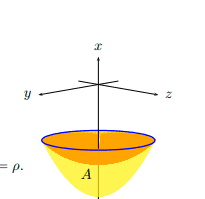My question is the following:
I want to do the integration by strate and here is my proposition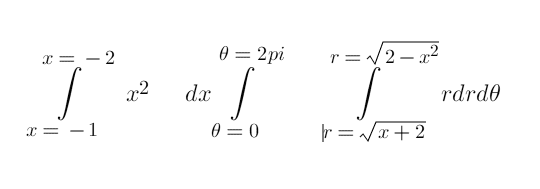is that even correct?
I would like to know if there is cases when switching between the two methods of integration is not possible?

•Delta2

Staff Emeritus
Homework Helper
I've never heard of "integration by strate." Could you explain what this means?

••Delta2 and Amaelle
Amaelle
Certainly look at the image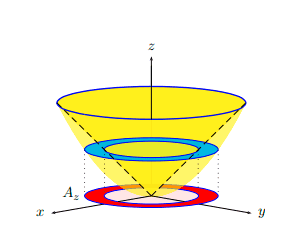it means doing the integration by rings and then integrating by hight

Staff Emeritus
Homework Helper
Oh, okay. In the US, I think that technique is referred to as the disc method.

•Delta2
Staff Emeritus
Homework Helper
You should be able to use that method, but you're going to have to break the integral up into two integrals, one from ##x=-2## to ##x=-\sqrt{2}## and one from ##x=-\sqrt{2}## to ##x=-1##.

••Delta2 and BvU
Amaelle
thank you very much , indeed I used your insights to solve the question under the disk method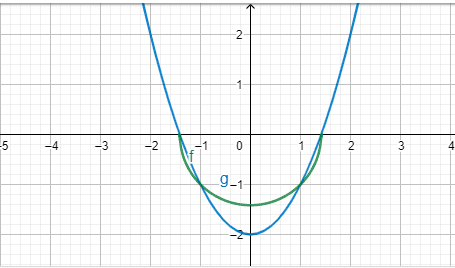but the only way I could find out was to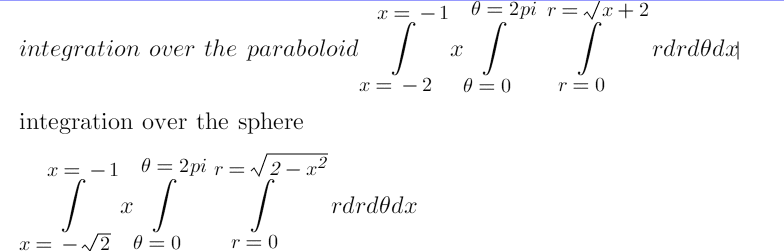and then substructing the sphere from the paraboloid
I would be very grateful if you could elaborate more about the setting of your method and why you need to split the integration this way?
thank you!

•Delta2
Staff Emeritus
Homework Helper
To keep it simple, I'm going to consider volume integrals but the same ideas apply to your problem.

When you do the integrals over ##r## and ##\theta##, you end up with an integral of the form
$$\int_{x_1}^{x_2} \pi r^2 \,dx$$ where we can identify ##\pi r^2## as the area of the disk and ##dV = \pi r^2\,dx## as the volume of the infinitesimal slice. In this particular problem, from ##x=-2## to ##x=-\sqrt{2}##, the volume is given by an integral of that form. However, from ##x=-\sqrt{2}## to ##x=-1##, you have a disk with a hole in the middle with area ##\pi(r_{\rm outer}^2-r_{\rm inner}^2)##. Hence, the volume of that portion is given by
$$\int_{x_1}^{x_2} \pi (r_{\rm outer}^2-r_{\rm inner}^2) \,dx.$$ Because you have two different integrands, you need to do the integrals for each range separately.

Note that you can manipulate your integrals similarly:
\begin{align*}
I &= \int_{-2}^{-1} \int_0^{2\pi} \int_0^{\sqrt{2-x^2}} xr\,dr\,d\theta\,dx
-\int_{-\sqrt 2}^{-1} \int_0^{2\pi} \int_0^{\sqrt{x+2}} xr\,dr\,d\theta\,dx \\
&= \int_{-2}^{-\sqrt{2}} \int_0^{2\pi} \int_0^{\sqrt{2-x^2}} xr\,dr\,d\theta\,dx
+\int_{-\sqrt{2}}^{-1} \int_0^{2\pi} \int_0^{\sqrt{2-x^2}} xr\,dr\,d\theta\,dx
-\int_{-\sqrt 2}^{-1} \int_0^{2\pi} \int_0^{\sqrt{x+2}} xr\,dr\,d\theta\,dx \\
&= \int_{-2}^{-\sqrt{2}} \int_0^{2\pi} \int_0^{\sqrt{2-x^2}} xr\,dr\,d\theta\,dx
+\int_{-\sqrt{2}}^{-1} \int_0^{2\pi} \int_{\sqrt{x+2}}^{\sqrt{2-x^2}} xr\,dr\,d\theta\,dx
\end{align*} The limits on the ##r## integral are different for ##x=-2## to ##x=-\sqrt{2}## and for ##x=-\sqrt{2}## to ##x=-1##.

•Amaelle
Amaelle
thanks a million!